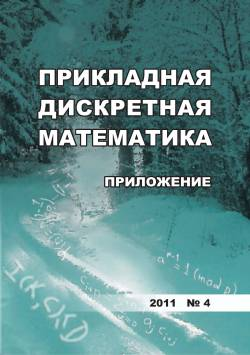# Свойства объединенных булевых функций квадратичных функций APN

For a function F : Fn ^ Fn, it is defined the associated Boolean function yf in 2n variables as follows: yf(a, b) = 1 if a = 0 and equation F(ж) + F(x + a) = b has solutions. A vectorial Boolean function F from Fn to Fn is called almost perfect nonlinear (APN) if equation F(ж) + F(ж + a) = b has at most 2 solutions for all vectors a, b Е Fn, where a is nonzero. In case when F is a quadratic APN function its associated function has the form yf(a, b) = (a) · b + (a) + 1 for appropriate functions \$F : Fn ^ Fn and : Fn ^ F2. We study properties of functions \$F and , in particular their degrees.

## Ключевые слова

APN functions, associated Boolean functions, differential equivalence

## Авторы

 ФИО Организация Дополнительно E-mail Городилова Анастасия Александровна Институт математики им. С. Л. Соболева СО РАН; Новосибирский государственный университет кандидат физико-математических наук, научный сотрудник; старший преподаватель gorodilova@math.nsc.ru
Всего: 1

## Ссылки

Nyberg K. Differentially uniform mappings for cryptography. EUROCRYPT'93, LNCS, 1994, vol. 765, pp. 55-64.
Glukhov M. M. O priblizhenii diskretnykh funktsiy lineynymi funktsiyami [On the approximation of discrete functions by linear functions]. Matematicheskie Voprosy Kriptografii, 2016, vol. 7, no. 4, pp. 29-50. (in Russian)
Carlet C., Charpin P., and Zinoviev V. Codes, bent functions and permutations suitable for DES-like cryptosystems. Designs, Codes and Cryptography, 1998, vol.15, iss.2, pp. 125-156.
Gorodilova A. On the differential equivalence of APN functions. Cryptography and Communications, 2019. https://link.springer.com/article/10.1007/ s12095-018-0329-y.
Boura C., Canteaut A., Jean J., and Suder V. Two notions of differential equivalence on S-boxes. Designs, Codes and Cryptography, 2019, vol. 87, iss. 2-3, pp. 185-202.
Gorodilova А. Lineynyy spektr kvadratichnykh APN-funktsiy [The linear spectrum of quadratic APN functions]. Prikladnaya Diskretnaya Matematika, 2016, no4(34), pp. 5-16. (in Russian)